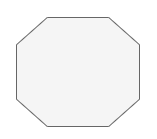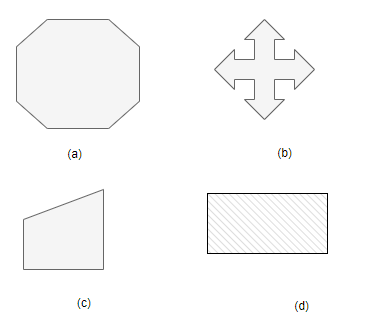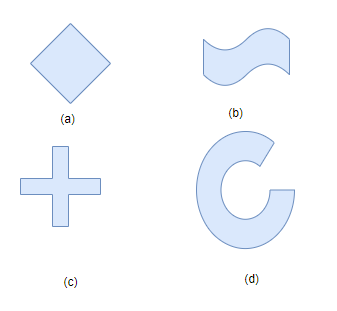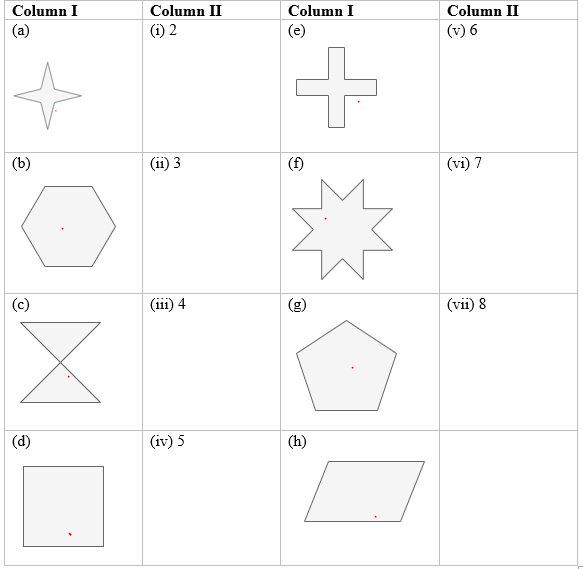# Symmetry Questions

In this page we have Important Questions Class 7 Maths Chapter 14: Symmetry . Hope you like them and do not forget to like , social share and comment at the end of the page.

## Fill in the blanks

Question 1.
(i) A figure has __________ symmetry if there is a line about which the figure may be folded so that the two parts of the figure coincide.
(ii) A regular pentagon has ____________ line of symmetry.
(iii) A figure has ____________ symmetry if after a rotation through a certain angle. The figure looks exactly the same.
(iv)A __________ triangle has no lines of symmetry
(v) Each regular polygon has as many lines of symmetry as it has _____________.
(vi) The concept of line symmetry is closely related to __________ reflection. (vii)The quadrilateral that has four lines of symmetry and order-four rotational symmetry is a _______________.
(viii) The angle of rotational symmetry for letter S is ________________.
(ix) A line segment is symmetrical about its _______________________________.
(x) Rotation turns an object about a fixed point. The fixed point is called ________________.
(xi)Each of the letters H, N, S and Z has a rotational symmetry of order __________.
(xii) The line of symmetry of an isosceles triangle is its ______________________ from the vertex having the equal sides.

(i) Line
(ii) 5
(iii) rotational
(iv)Scalene
(v) number of sides
(vi) mirror
(vii) square
(viii)180°, 360°
(ix) perpendicular bisector
(x) center of rotation
(xi) 2
(xii) Median or altitude

## State true or false

Question 2.
(i) When an object rotates, its shape changes.
(ii) if a shape possess rotational symmetry, it will surely have line of symmetry.
(iii) We can not have a rotational symmetry of order more than 1 whose angle of rotation is 23°
(iv) A pentagon which has more than one line of symmetry must be regular.
(v)A regular hexagon has six lines of symmetry.
(vi) A figure having two or more lines of symmetry has rotational symmetry of order more than 1.
(vii) The wheel of a bicycle can rotate both ways clockwise and anticlockwise.
(viii) The centre of rotation in a parallelogram is the point of intersection of its diagonals.
(ix) An equilateral triangle has six lines of symmetry
(x) Rotation turns an object about a fixed point which is known as centre of rotation.

(i) False
(ii) False
(iii) True
(iv) True
(v) True
(vi) True
(vii)True
(viii) true
(ix) False
(x) True

## Multiple Choice Questions

Question 3.
In the word "MATHS" which of the following pairs of letters shows rotational symmetry
(a) M and T
(b) H and S
(c) A and S
(d) T and S
Question 4.
How many letters in the word SNAIL have line symmetry?
(a) 2
(b) 3
(c) 4
(d) 1
Question 5.
Order of rotational symmetry of(a) 6
(b) 4
(c) 8
(d) 2
Question 6.
Which of the following letters of English alphabets have more than 2 lines of symmetry?
(a) Z
(b) O
(c) C
(d) H
Question 7.
Which of the following figures do not have line symmetry?Question 8.
Which of the following figures do not have rotational symmetry?3. (b)
4. (a)
5. (c)
6. (b)
7. (c)
8. (d)

## Match the Column

Question 9.
Match order of rotational symmetry of these shapes:(a) -> (iii)
(b) -> (v)
(c) -> (i)
(d) -> (iii)
(e) -> (iii)
(f) ->(vii)
(g) -> (iv)
(h) -> (i)

Assignments